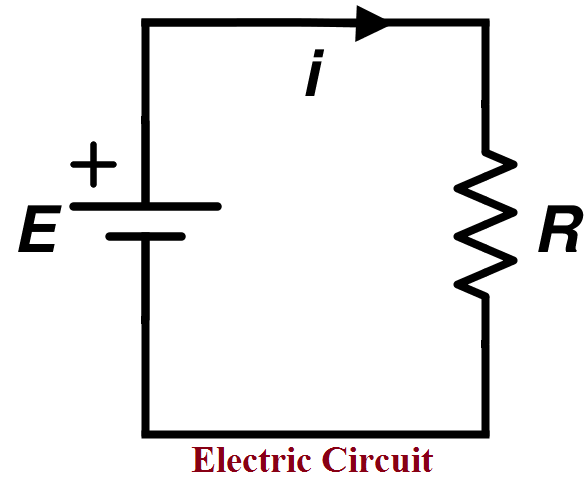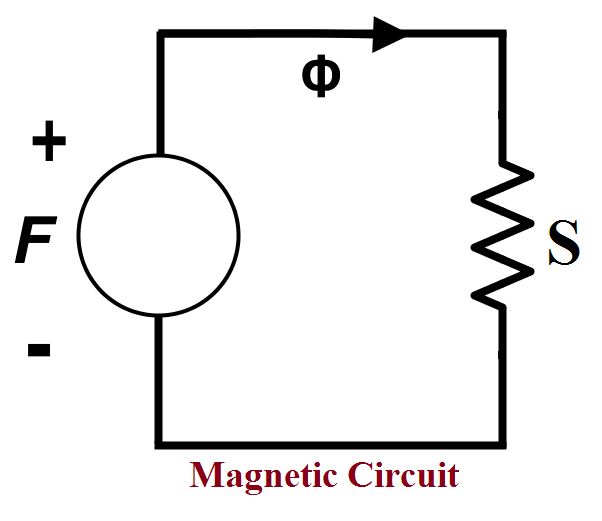# Top 7 Difference between Resistance and ReluctanceIn the earlier difference between electrical and magnetic circuit tutorial, we have learned about electromagnetic opposition quantities (which opposes the flow of electric current in the circuit) such as resistance and reluctance.

These two quantities are having same opposition property. But, both have different specifications and used in different circuits.

### Difference between Resistance and Reluctance

Resistance vs Reluctance in tabular format.

 Sr. No. Content Resistance Reluctance 1 Definition The property of the device which opposes the flowing current in the electrical circuit is known as Resistance. The property of the device which opposes the flowing magnetic flux in a magnetic circuit is known as Reluctance. 2 Representation The resistance is represented by R. The reluctance is represented by S. 3 Used (Requirement) It is used in an electrical and Electronic circuit. It is used in a magnetic circuit. 4 Formula Resistance is calculated by using formula- ```R = ( EMF / I ) or R = ( ρ l / a )``` Where, EMF – Electromotive Force I – Electric Current ρ – Resistivity l – Length of the conductor a – Area of the material Reluctance is calculated by using formula- ```S = ( MMF /‍ Φ ) or S = ( l / µ a )``` Where, MMF – Magnetomotive Force Φ – Magnetic flux l – Length of the conductor µ – Magnetic permeability a – Area of the material 5 Unit Resistance is measured in Ohm (Ω). Reluctance is measured in Ampere-turns/Weber (AT / Wb). 6 Property (Function) The primary function of resistance is to limit the flow of electric current in an electrical circuit or system. The primary function of reluctance is to limit the flow of magnetic flux in the magnetic circuit.

Circuit Diagram:Figure: Resistance in Electrical CircuitFigure: Reluctance in Magnetic Circuit

Resistance Calculator formula:

You can calculate the resistance (R) of the circuit from the current (I) and voltage (V) values.

```Resistance = (Voltage / Current)  or

Resistance = (Electromotive Force / Current )```

Here is a simple calculator you can use to find the resistance.

Reluctance Calculator formula:

You can calculate the reluctance (S) of the circuit from the magnetic flux (Φ) and magnetomotive force (MMF) values.

`Reluctance = (Magnetomotive Force / Magnetic Flux )`

Here is a simple calculator you can use to find the reluctance.

These are very simple points to understand these points about the difference between resistance and reluctance. If you have any doubts, comment below.

More related Topic:

If You Appreciate What I Do Here On DipsLab, You Should Consider:

DipsLab is the fastest growing and most trusted community site for Electrical and Electronics Engineers. All the published articles are available FREELY to all.

If you like what you are reading, please consider buying me a coffee ( or 2 ) as a token of appreciation.

This will help me to continue my services and pay the bills.I’m thankful for your never-ending support.

### 2 thoughts on “Top 7 Difference between Resistance and Reluctance”

1.Well explained and summarised notes.

•